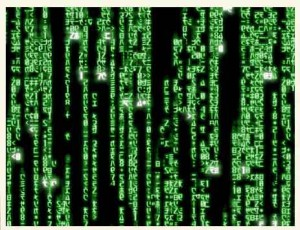Here are some of my favorite nonlinear formulas in linear algebra. Most of them play a crucial role in mathematics such as in numerical analysis, in statistics, and in random matrix theory. In what follows, the letters ${A,B,C,D,U,V,W,M}$ denote matrices while the letters ${u,v}$ denote column vectors (special matrices).

• The blockwise inversion formula states that as soon as both sides make sense

$\begin{pmatrix} A & B \\ C & D \end{pmatrix}^{-1} = \begin{pmatrix} A^{-1}+A^{-1}B(D-CA^{-1}B)^{-1}CA^{-1} & -A^{-1}B(D-CA^{-1}B)^{-1} \\ -(D-CA^{-1}B)^{-1}CA^{-1} & (D-CA^{-1}B)^{-1} \end{pmatrix}$

The matrix ${D-CA^{-1}B}$ is the Schur complement of ${A}$ in the ${(A,B,C,D)}$ block-matrix above. In particular for every ${n\times n}$ matrix ${M}$ and any non empty ${I\subset\{1,\ldots,n\}}$ we have

$(M^{-1})_{I,I} = (M_{I,I} – M_{I,I^c}(M_{I^c ,I^c})^{-1}M_{I^c,I})^{-1} .$

The Schur complement is at the heart of the Gaussian linear model in statistics. We have already discussed the role of the Schur complement in the geometry of positive definite matrices, and an application to the computation of the Wasserstein distance between two Gaussians.

• The Woodbury matrix identity states that as soon as both sides make sense we have

$(A+UCV)^{-1}=A^{-1}-A^{-1}U(C^{-1}+VA^{-1}U)^{-1}VA^{-1}$

This contains as a special case the Sherman-Morrison formula

$(A+uv^\top)^{-1}=A^{-1}-\frac{A^{-1}uv^\top A^{-1}}{1+v^\top A^{-1}u}$

These formulas are at the heart of the Gaussian Kalman filter in engineering and the Cauchy-Stieltjes trace-resolvent method in random matrix theory

• The binomial inverse theorem provides an alternative to the Woodbury formula

$(A+UCV)^{-1} =A^{-1} – A^{-1}UC(C+CVA^{-1}UC)^{-1}CVA^{-1}$

• The matrix determinant lemma states that as soon as both sides make sense we have the identity

$\det(A+UWV^\top)=\det(W^{-1}+V^\top A^{-1}U)\det(W)\det(A)$

This contains as a special case the Sylvester determinant theorem

$\det(I_n+AB)=\det(I_m+BA)$

where ${I_d}$ is the ${d\times d}$ identity matrix, and also the formula

$\det(A+uv^\top)=(1+v^\top A^{-1}u)\det(A).$

• The resolvent formula states that for any square matrices ${A}$ and ${B}$ and any ${z\not\in\mathrm{spec}(A)\cup\mathrm{spec}(B)}$,

$(A-zI)^{-1}-(B-zI)^{-1} = (A-zI)^{-1}(B-A)(B-zI)^{-1}.$

It follows immediatly from the identity ${(B-zI)-(A-zI)=B-A}$.

If you don’t know these formulas, they know you (everywhere in numerical software packages).

Last Updated on

This site uses Akismet to reduce spam. Learn how your comment data is processed.

Syntax · Style · .